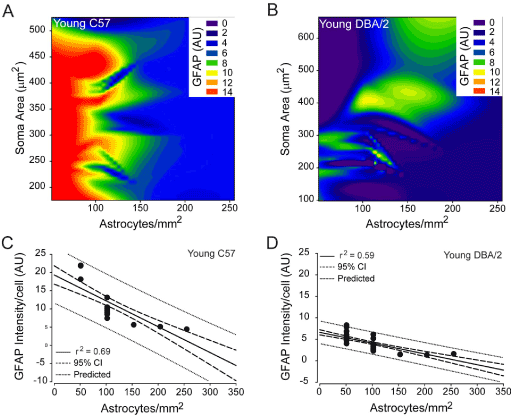Figure 6: Astrocyte density reliably predicts GFAP expression by spatially-coincident astrocytes in young C57 and young DBA/2 retina. (A and B) Contour plot depicting the relationships between mean GFAP intensity/cell (color bar; arbitrary units), astrocyte density (x-axis; astrocytes/mm2) and soma area (y-axis; μm2) in young C57 (A) and young DBA/2 (B) retina. (C) Regression graph of the negative correlation between astrocyte density and mean GFAP expression/cell in young C57 retina plotted as astrocytes/mm2 (x-axis) versus arbitrary units of intensity (y-axis). Solid lines indicate regression line based on r2 value. Dashed lines indicate the 95% confidence interval. Dotted lines indicate predicted values based on r2 and 95% confidence. (D) Regression graph of the negative correlation between astrocyte density and mean GFAP expression/cell in young DBA/2 retina plotted as astrocytes/mm2 (x-axis) versus arbitrary units of intensity (y-axis). Solid lines indicate regression line based on r2 value. Dashed lines indicate the 95% confidence interval. Dotted lines indicate predicted values based on r2 and 95% confidence.